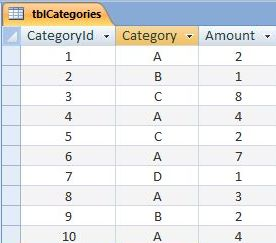## Friday, 5 August 2011

### Using the DSum Function

Today we are going to look at using the DSum Function within a Calculated Control to produce a Total Amount. The DSum Function works in a similar way to the DLookUp function which I wrote about in my last post. Both functions pass an identical set of parameters in their syntax. However, instead of looking up a field value in a table or query, the DSum Function calculates the sum of values contained in a particular field of a specified table.

So lets take a look at the syntax of the DSum Function:

`=DSum("fieldName", "tableName", "criteria")`

The first parameter we pass is the name of the field containing the group of values that we wish to add together as a sum.  The second parameter is the name of the table or query, and the third parameter is an optional criteria used to restrict the group of records upon which the calculation is to be made.  All the parameters are passed as strings - hence the quotation marks.

So, for example, if we had a field called Amount, and a table called tblCategories, our expression would look like this:

`=DSum("Amount", "tblCategories", "fldCategory='A'")`

The final parameter is the criteria string. In this example the criteria restricts the calculation to records containing the value 'A' in the fldCategory field.  As such this function would produce a Total Amount for all records allocated the category value 'A'.

So lets put all this into practice.  In figure one below I have created the tblCategories table and populated it with values.  There are four different categories A,B,C and D.  Each instance of a category has an amount value associated with it.Figure 1: The Categories Table (tblCategories)

I then created a form with four Calculated Controls.  I used the DSum Function in each control to calculate a Total Amount for each category.Figure 2: The DSum Function within Calculated Form Controls.

And here are the results:Figure 2: Results of the DSum Function.

1.WHAT IF THERE ARE 100 CATEGORIES ?

2.Hi Raogururaj

Thanks for your comment. It is an excellent question:)

The above exercise was something I set up to illustrate how the DSum Function works. It has limited practical value in itself - especially if as you say, there were 100 categories.

The best way to deal with that scenario would be to set up a query.

Click the QUERY DESIGN icon from the CREATE ribbon. Select the CATEGORY and AMOUNT fields from tblCategories, and place them on the Query Design Grid. Then click the TOTALS icon from the SHOW/HIDE group of the DESIGN ribbon. This reveals an extra TOTALS row on the Grid. Set the TOTALS value for the CATEGORY field to GROUP BY, and the TOTALS value for AMOUNT field to SUM.

When you run the query, each Category is displayed in its own row, along with the total amount for each category. The layout of data is the same as the form version which uses the DSum Calulated controls in the above post.

Hope this helps,

Justin

3.Hi Justin,

Thanks for the info. I am trying DSUM out on a report but I keep getting an error. Does this look right?
=DSum("Price","Sales","Payment Method = 'cash'")

Thanks,
Hector

1.Hi Hector

The syntax itself looks ok. Can you confirm that PRICE is the field name, SALES is the table name, and that the criteria is searching for a text value called CASH, from a field name called PAYMENT METHOD? If so, please can you tell me what error message you are getting?

2.I think the issue might be the Payment Method field name. Since it has a space in the name it will need brackets inside the string:

=DSum("Price","Sales","[Payment Method] = 'cash'")

4.Is it possible to set the recordset to the form ex: me.Recordset

I am trying to use DSum to calculate a field with a particular criteria on a form after a filter for month is applied to the form's recordset

=DSum("[FieldName]","Me.Recordset","Criteria")
I have also tried
=DSum("[FieldName]","[forms]![FormName]![FieldName]","Criteria")

The criteria specified works when the query on which the form is based is specified, but then it returns a total sum for that field

1.Hi Terry
Are you using the DSum function within a VBA project? If so you can refer to the Recordset.Name property of the underlying form. For example:

myTotal = DSum("amount", Me.Recordset.Name, "category = 'A'")